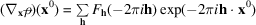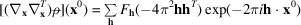International
Tables for
Crystallography
Volume B
Reciprocal space
Edited by U. Shmueli

International Tables for Crystallography (2010). Vol. B, ch. 1.3, p. 96   | 1 | 2 |

## Section 1.3.4.4.7.2. Booth's differential Fourier syntheses

G. Bricognea

aGlobal Phasing Ltd, Sheraton House, Suites 14–16, Castle Park, Cambridge CB3 0AX, England, and LURE, Bâtiment 209D, Université Paris-Sud, 91405 Orsay, France

#### 1.3.4.4.7.2. Booth's differential Fourier syntheses

| top | pdf |

It was the use of Fourier syntheses in the completion of trial structures which provided the incentive to find methods for computing 2D and 3D syntheses efficiently, and led to the Beevers–Lipson strips. The limited accuracy of the latter caused the estimated positions of atoms (identified as peaks in the maps) to be somewhat in error. Methods were therefore sought to improve the accuracy with which the coordinates of the electron-density maxima could be determined. The naive method of peak-shape analysis from densities recalculated on agrid using high-accuracy trigonometric tables entailed 27 summations per atom.

Booth (1946a)suggested examining the rapidly varying derivatives of the electron density rather than its slowly varying values. Ifthen the gradient vectorofatcan be calculated by means of three Fourier summations from thevector of Fourier coefficientsSimilarly, the Hessian matrix ofatcan be calculated by six Fourier summations from the unique elements of the symmetric matrix of Fourier coefficients:The scalar maps giving the components of the gradient and Hessian matrix ofwill be called differential syntheses of 1st order and 2nd order respectively. Ifis approximately but not exactly a maximum of, then the Newton–Raphson estimate of the true maximumis given by:This calculation requires only nine accurate Fourier summations (instead of 27), and this number is further reduced to four if the peak is assumed to be spherically symmetrical.

The resulting positions are affected by series-termination errors in the differential syntheses. Booth (1945c, 1946c)proposed a `back-shift correction' to eliminate them, and extended this treatment to the acentric case (Booth, 1946b). He cautioned against the use of an artificial temperature factor to fight series-termination errors (Brill et al., 1939), as this could be shown to introduce coordinate errors by causing overlap between atoms (Booth, 1946c, 1947a,b).

Cruickshank was able to derive estimates for the standard uncertainties of the atomic coordinates obtained in this way (Cox & Cruickshank, 1948; Cruickshank, 1949a,b) and to show that they agreed with those provided by the least-squares method.

The calculation of differential Fourier syntheses was incorporated into the crystallographic programs of Ahmed & Cruickshank (1953b)and of Sparks et al. (1956).

### References

Ahmed, F. R. & Cruickshank, D. W. J. (1953b). Crystallographic calculations on the Manchester University electronic digital computer (Mark II). Acta Cryst. 6, 765–769.
Booth, A. D. (1945c). Accuracy of atomic co-ordinates derived from Fourier synthesis. Nature (London), 156, 51–52.
Booth, A. D. (1946a). A differential Fourier method for refining atomic parameters in crystal structure analysis. Trans. Faraday Soc. 42, 444–448.
Booth, A. D. (1946b). The simultaneous differential refinement of co-ordinates and phase angles in X-ray Fourier synthesis. Trans. Faraday Soc. 42, 617–619.
Booth, A. D. (1946c). The accuracy of atomic co-ordinates derived from Fourier series in X-ray structure analysis. Proc. R. Soc. London Ser. A, 188, 77–92.
Booth, A. D. (1947a). The accuracy of atomic co-ordinates derived from Fourier series in X-ray structure analysis. III. Proc. R. Soc. London Ser. A, 190, 482–489.
Booth, A. D. (1947b). The accuracy of atomic co-ordinates derived from Fourier series in X-ray structure analysis. IV. The two-dimensional projection of oxalic acid. Proc. R. Soc. London Ser. A, 190, 490–496.
Brill, R., Grimm, H., Hermann, C. & Peters, C. (1939). Anwendung der röntgenographischen Fourieranalyse auf Fragen den chemischen Bindung. Ann. Phys. (Leipzig), 34, 393–445.
Cox, E. G. & Cruickshank, D. W. J. (1948). The accuracy of electron-density maps in X-ray structure analysis. Acta Cryst. 1, 92–93.
Cruickshank, D. W. J. (1949a). The accuracy of electron-density maps in X-ray analysis with special reference to dibenzyl. Acta Cryst. 2, 65–82.
Cruickshank, D. W. J. (1949b). The accuracy of atomic co-ordinates derived by least-squares or Fourier methods. Acta Cryst. 2, 154–157.
Sparks, R. A., Prosen, R. J., Kruse, F. H. & Trueblood, K. N. (1956). Crystallographic calculations on the high-speed digital computer SWAC. Acta Cryst. 9, 350–358.